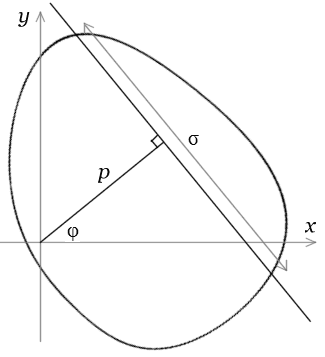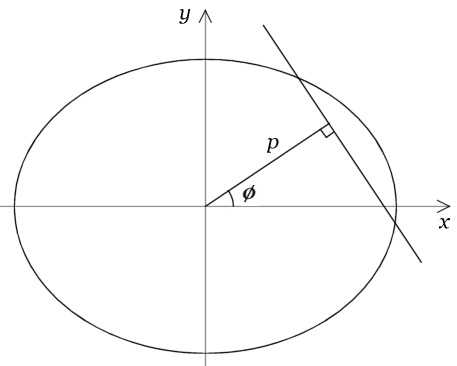Probability moments for ellipse and rectangle

Let K be a finite plane convex region. In working out the expected number of alignments in a random set of points, with independent uniform distributions in K, we need the moments of the p.d.f. for |P1P2|, the distance between two points P1 and P2 with independent uniform distributions in K. Denote by Mn the nth moment, i.e. the mean value of |P1P2|n.

With a strip criterion, the expected number of r-point alignments (r3) typically depends on Mr2, so that all positive moments are needed. With an angle criterion, the expected number of r-point alignments typically depends on M2r4, so that only even moments are needed. In the formulae below, the form of Mn differs for odd and even n.

The lists below give the first few values of Mn for K = ellipse and rectangle, with circle and square listed separately for convenience. There is then a description of how the moments can be calculated for these K.

Circle

For a circle with radius a :

 M1= 128a 45π
 M2= a2
 M3= 2048a3 525π
 M4= 5a4 3
 M5= 16384a5 2205π
 M6= 7a6 2

Ellipse

For an ellipse with semi-major axis a and eccentricity e :

Here K(e) and E(e) are complete elliptic integrals (see below).

 M1 = 256a E(e) 45π2
 M1 = 128a ( 1− 1 e2− 3 e4− 5 e6− 175 e8− 441 e10−… ) 45π 4 64 256 16384 65536
 M2 = ( 1− 1 e2 ) 2
 M3 = 4096a3 ( (4−2e2)E(e)−(1−e2)K(e) ) 1575π2
 M3 = 2048a3 ( 1− 3 e2+ 9 e4+ 5 e6+ 105 e8+ 189 e10+… ) 525π 4 64 256 16384 65536
 M4 = 5a4 ( 1− e2+ 3 e4 ) 3 8
 M5 = 32768a3 ( (23−23e2+8e4)E(e)−4(1−e2)(2−e2)K(e) ) 33075π2
 M5 = 16384a5 ( 1− 5 e2+ 45 e4− 25 e6− 175 e8− 189 e10−… ) 2205π 4 64 256 16384 65536
 M6 = 7a6 ( 1− 3 e2+ 9 e4− 5 e6 ) 2 2 8 16

Square

For a square with side a:

 M1= a ( 2+√2+5ln(√2+1) ) 15
 M2= a2 3
 M3= a3 ( 8+17√2+21ln(√2+1) ) 210
 M4= 17a4 90
 M5= a5 ( 16+73√2+45ln(√2+1) ) 1008
 M6= 29a6 210

Rectangle

For a rectangle with sides a and b:

Let d=(a2+b2), and let

 Ln= an+1 ln ( d+b ) + bn+1 ln ( d+a ) b a a b

Then

 M1= 1 ( 10d− 2(d 5−a5−b5) +5L1 ) 30 a2b2
 M2= 1 d 2 6
 M3= 1 ( 49d 3− 8(d 7−a7−b7) +21L3 ) 420 a2b2
 M4= 1 ( 6a4+5a2b2+6b4 ) 90
 M5= 1 ( 3d (41a4+52a2b2+41b4)− 16(d 9−a9−b9) +45L5 ) 2016 a2b2
 M6= 1 ( 15a6+14a4b2+14a2b4+15a6 ) 420

A theorem of Santaló

A theorem given by Santaló [Ref. 1] is useful in calculating the moments.Let P1P2=r. Santaló defines

 Jn= ∫ rndP1dP2 . P1 ,P2∈K

Let G be a variable line defined by coordinates (p,φ) (Fig. 1b), and if G meets K let σ be the length of the chord so defined. Santaló defines

 In= ∫ σndG , G∩K≠∅

which we may write as

 In= ∫ ∞ ∫ 2π σndpdφ , p=0 φ=0

taking σ=0 if G does not meet K. Santaló proves that

 Jn= 2 In+3 . (n+2)(n+3)

Now let |K| be the area of K and let Mn be the nth moment of the p.d.f. of r. Clearly

 Mn= Jn |K|2

and consequently

 Mn= 2 In+3 . (n+2)(n+3) |K|2

It may be easier to evaluate In+3 and apply this formula, than to evaluate Mn directly.

Moments for an ellipseWe evaluate Mn for an ellipse from Santaló’s In+3, as above.

Let the equation of the ellipse be x2/a2+y2/b2=1, and let e be the eccentricity, so that b2=a2(1e2). Setting

 q(φ)=a(1−e2sin2φ)1/2 ,

we find by a routine calculation that the line (p,φ) meets the ellipse iff |p|q(φ), and then

 σ=2abq(φ)−2 (q(φ)2−p2)1/2 .

Putting p = q(φ)sinθ gives

 In= ∫ π/2 ∫ 2π [2abq(φ)−2]n [q(φ)cosθ]n+1dθdφ = 2nCn+1abn ∫ π/2 (1−e2sin2φ)−(n−1)/2 , θ=0 φ=0 0

where

 Cm= ∫ π/2 cosmθdθ , so that C0=π/2, C1=1, Cm=Cm−2(m−1)/m  (m=2,3,…). 0

Combining, and noting that the integral from 0 to is 4 times the integral from 0 to π/2, we get

 Mn= 2n+6Cn+4 bn+1 ∫ π/2 (1−e2sin2φ)−(n+2)/2dφ. (n+2)(n+3) π2a 0

The integral here (call it An) can be done for a particular e by numerical integration. Alternatively, it can be solved explicitly as follows. To bring An into the form tabulated by Gradshteyn & Ryzhik [Ref. 2], define

 θ=π−2φ, c=(1−e2)1/2, ƒ= 1−c . 1+c

Then

 An= ∫ π/2 (1−e2sin2φ)−(n+2)/2=2n+1(1−c)−(n+2) ∫ π (1−2ƒcosθ+ƒ2)−(n+2)/2 . 0 0

Case of even n.  Let n=2m. From item 3.616.2 of [Ref. 2],

 An=2n+1(1+c)−(n+2)×π(1−ƒ2)−(m+1) ∑ m (m+k)! uk , k=0 k!2(m−k)!

where u=ƒ2/(1ƒ2)=(1c)2/4c. Simplifying slightly,

 An=π(2c)−(2m+1) ∑ m (m+k)! (1−c)2k(4c)m−k . k=0 k!2(m−k)!

One checks empirically (I have no proof yet) that

 ∑ m (m+k)! (1−c)2k(4c)m−k= ∑ m (2k)! (2m−2k)! c2k= ∑ m (2k)!m! 4m−k(−e2)k . k=0 k!2(m−k)! k=0 k!2 (m−k)!2 k=0 k!3(m−k)!

For even n=2m we conclude

 Mn= 2n+4 ⋅ n+1 ⋅ n−1 ⋅…⋅ 1 an ∑ m (2k)!m! (−e2/4)k , (n+2)(n+4) n+2 n 2 k=0 k!3(m−k)!

where for a circle (e = 0) the sum equals 1.

Case of odd n.  Let n = 2m – 1. From item 3.617 of [Ref. 2],

 An= 2n+1 2 Fm ( 2ƒ1/2 ) , (1+c)n+2 (1+ƒ)n+2 1+ƒ

where the functions Fm are defined below. Since (1+c)(1+ƒ)=2 and 1/2(1+ƒ)=e, we have

 Mn= 2n+6Cn+4 an (1−e2)m Fm(e) . (n+2)(n+3) π2

We now define the Fm as in [Ref. 2]. F0 is the complete elliptic integral of the first kind, given by

 F0(e)= K(e)= ∫ π/2 (1−e2sin2θ)−1/2dθ= π ( 1+ 12 e2+ 12⋅32 e4+ 12⋅32⋅52 e6+… ) 0 2 22 22⋅42 22⋅42⋅62

and the Fm satisfy the recurrence relation

 Fm+1(e)=Fm(e)+ e dFm(e) (m=0,1,2,…). 2m+1 de

In our case it is convenient to define Gm(e)=(2/π)(1e2)mFm(e). The recurrence relation for the Gm is

 Gm+1(e)=Gm(e)+ 1 ( e(1−e2) dGm(e) −e2Gm(e) ) (m=0,1,2,…). 2m+1 de

If we introduce as in [Ref. 2] the complete elliptic integral of the second kind, given by

 E(e)= ∫ π/2 (1−e2sin2θ)1/2dθ= π ( 1− 1 e2− 12⋅3 e4− 12⋅32⋅5 e6−… ) 0 2 22 22⋅42 22⋅42⋅62

then the derivatives

 dK(e) = E(e) − K(e) , dE(e) = E(e)− K(e) , de e(1−e2) e de e

combined with the recurrence relation for Fm, lead to

 G1(e)= 2 (1−e2) F1(e)= 2 E(e) , π π
 G2(e)= 2 (1−e2)2 F2(e)= 2 ( (4−2e2)E(e)−(1−e2)K(e) ) , π 3π
 G3(e)= 2 (1−e2)3 F3(e)= 2 ( (23−23e2+8e4)E(e)−4(1−e2)(2−e2)K(e) ) , π 15π

and so on (the working becomes laborious). Alternatively, the recurrence relation for Gm leads to

 Gm(e)=1− 1 (2m−1)e2+ 1.3 (2m−1)(2m−3)e4− 1⋅3⋅5 (2m−1)(2m−3)(2m−5)e6+… . 22 22⋅42 22⋅42⋅62

For odd n=2m1 we conclude

 Mn= 2n+4 ⋅ n+1 ⋅ n−1 … 2 ⋅ 2 an Gm(e) , (n+2)(n+4) n+2 n 3 π

where for a circle Gm(0) = 1.

It is possible to combine the results for even and odd n into a single formula, but this is less convenient for applications.

Moments for a rectangleWe evaluate Mn for a rectangle from Santaló’s In+3, as above.

Let the diagonal of the rectangle be d, and let the sides be a=dco, b=dsi. It suffices to consider 0φπ/2.

First suppose 0φπ/2γ. Define p1=(d/2)cos(γ+φ) , p2=(d/2)cos(γφ)S. The chord length σ for the line (p,φ) is given by

 σ=bsecφ if  0≤p≤p1 σ=bsecφ×(p2−p)/(p2−p1) if  p1≤p≤p2 0 if  p>p2

from which we easily find

 ∫ ∞ σndp=(d sinγ secφ)n ( d cos(γ+φ) + d sinγ sinφ ) . 0 2 n+1

Similarly, for π/2γφπ/2 we find

 ∫ ∞ σndp=(d cosγ cosecφ)n ( − d cos(γ+φ) + d cosγ cosφ ) , 0 2 n+1

which has the same form as the first integral if we put  γ=π/2γ′φ=π/2φ′.

Integrating over φ=0 to we find for n>0 that

 In=2d n+1 sinnγ cosγ ∫ π/2−γ secn−1φdφ− 2 d n+1(sin2γ−sinn+1γ)+ comp, 0 n+1

where “comp” is obtained by putting π/2γ in place of γ. Recall that if

 Sm(θ)= ∫ θ secmφdφ 0

for 0θπ/2 and integer m>0, then

 S1(θ)=ln(secθ+tanθ), S2(θ)=tanθ,
 Sm(θ)= 1 tanθ secm−2θ+ m−2 Sm−2(θ) (m=3,4,…). m−1 m−1

Hence if we define

 U2= d ln ( d+b ) , U3=1, Um= 1 + m−3 ⋅ a2 Um−2 , b a m−2 m−2 d 2
 V2= d ln ( d+a ) , V3=1, Vm= 1 + m−3 ⋅ b2 Vm−2 , a b m−2 m−2 d 2

then the moments Mn are given by

 Mn= 4 ( d n (Un+3+Vn+3) − d  n+4−a n+4−b n+4 ) . (n+2)(n+3) (n+4)a2b2

For even n this expression reduces to a polynomial in a2,b2. Putting n=2m we have

 M2m=m! ∑ m a2(m−k) b2k . k=0 (2k+1) (2m−2k+1) (k+1)! (m−k+1)!

There does not seem to be a similar result for odd n.

References

  Luis A. Santaló, Integral Geometry and Geometric Probability (Vol. 1 of Encyclopedia of Mathematics and its Applications), Addison-Wesley, 1976, pp. 45–49.  I.S. Gradshteyn & I.M. Ryzhik, Table of Integrals, Series, and Products, 6th edn, Addison-Wesley, 2000, pp. 387–388.